• 下降迭代算法

千次阅读 2014-03-28 08:48:27
本文介绍下降迭代算法的概念、步骤和终止条件。 1. 为何需要迭代方法？  在无约束问题的极值条件中，我们讨论过极值的必要条件和充分条件。理论上讲，可以应用这些条件来求解相应的非线性规划问题的最优解。但在...

摘要：迭代方法是求解非线性规划问题的基本方法。利用迭代算法求解非线性规划问题的关键在于：一、构造每一轮的搜索方向；二、确定步长。本文介绍下降迭代算法的概念、步骤和终止条件。

1. 为何需要迭代方法？

在无约束问题的极值条件中，我们讨论过极值的必要条件和充分条件。理论上讲，可以应用这些条件来求解相应的非线性规划问题的最优解。但在实际问题中，可能会遇到以下问题：

1.     目标函数的导数不存在；
2.     导数存在，但为非线性方程组，求解困难甚至无解析解；
3.     问题为约束优化问题，不能简单套用无约束问题的极值条件。

与直接基于极值条件解析求解相对应的，是基于数值计算的迭代方法。事实上，迭代方法是求解非线性规划的更为一般的方法。我们在这里讨论极小值问题，其常用方法为下降迭代算法。

2. 下降迭代算法的概念

下降迭代算法主要包括两个概念：迭代与下降。

迭代  在优化计算中，迭代是指从已知点出发，依照某种规则（即算法）求出后继点，用 取代 ，然后重复以上过程，这样便会产生点列 和数列 。我们希望点列趋近于最优解，数列趋近于最优值。

下降  所谓下降，是指对某个函数，在每次迭代中，后继点的函数值都比原来的函数值有所减小。

设为某迭代算法第 k 轮的迭代点，为第 k+1 轮的迭代点，则它们之间的关系可以表示为

其中，，即为正实数，为向量方向上的单位向量。在已知的情况下求解只需要确定。可见，利用迭代算法求解非线性规划问题的关键在于：一、构造每一轮的搜索方向；二、确定最优步长。

3. 下降方向

通俗地讲，下降方向即为使函数值减小的自变量变化方向。但该理解不够严格，容易引起误解。其形式化定义如下：

下降方向  设，点,向量，若存在一个实数使得，都有

成立。则称在点处的下降方向。

4. 下降迭代算法的一般步骤

下降迭代算法的一般步骤可描述如下：

1. 选取初始点，令
2. 构造处的下降方向
3. 确定搜索步长使得目标函数值充分减小。注意，由于已知，是关于的一元函数。另外，尽管可以在每一步选取最优步长，即，但通常没有必要，因为求解最优步长可能会增加问题的复杂度；
4. 更新迭代点
5. 判断是否符合终止条件，若是，终止迭代；否则，返回步骤2。

5. 算法的终止条件

常用于判断算法终止条件的因素包括：1）自变量变化值； 2）目标函数变化值； 3）梯度值。具体包括以下几种情况：

1） 当自变量的改变值充分小时，即两次迭代的绝对误差或相对误差

或

时，迭代终止。

2）当目标函数的下降量充分小时，即相继的两次迭代的绝对误差或相对误差

或

时，算法终止。

3）当目标函数（假定目标函数可微）的梯度的模充分小（即目标函数的值接近于零）

时，算法终止。

展开全文非线性优化 极值 迭代 优化
• 这里写自定义目录标题梯度下降法算法详解新的改变功能快捷键合理的创建标题，有助于目录的生成如何改变文本的样式插入链接与图片如何插入一段漂亮的代码片生成一个适合你的列表创建一个表格设定内容居中、居左、居右...

应用情景

当我们有了一堆数据，需要用这堆数据构建模型时，首先需要确定模型中的变量，其次确定模型形式，最后需要确定模型中的参数。如构建一个多元线性回归模型，参数就是变量前的系数。

选择模型参数的标准是什么？并不统一。但一般需要构造一个关于参数 θ \theta 的损失函数 F ( θ , X ) F(\theta,X) （X实际为已知数，就是样本值），一般逻辑是，若真实值和模型预测值差距较大， F ( θ , X ) → a F(\theta,X)\to a ，若真实值和模型预测值差距较小， F ( θ , X ) → b F(\theta,X)\to b ，且 F ( θ , X ) ∈ ( a , b ) F(\theta,X)\in (a,b) 。（这里的数学表达可能并不准确，勿喷）

接下来要构造的问题是，求参数 θ \theta ，使 F ( θ , X ) F(\theta,X) m i n min 。这就是一个优化问题，需要选取一个优化算法。优化问题又分为有约束优化和无约束优化。本文仅讨论无约束优化，有约束优化放到以后再说。

另外，可以注意到，样本值的选取会影响 F ( θ , X ) F(\theta,X) 的形式，从而影响参数 θ \theta 的选取。例如，在训练一元线性回归模型中，若选取100个样本，则 F ( θ , X ) F(\theta,X) 中包含100个预测值和真实值之差的平方和；而若选取10个样本，则只包含10个项的平方和。对这两个函数取极值，一般会有很大的不同。因此，如何从样本中选取训练集来训练参数，也是一个重要问题。

为何不直接求解析解？

求解无约束优化问题有多种算法，如传统的公式解和数值优化。前者是通过代数方法解出方程理论解，是一个精确值；而数值优化方法求出的是一个近似值。这种方法又分为一阶优化算法（如梯度下降法）、二阶优化算法（如牛顿迭代法）、非梯度优化算法等。

为什么需要求近似值呢？因为有时候，需要解的函数太复杂，甚至不存在一个求解的公式。比如，伽罗瓦在群论中证明了，五次及以上多项式方程没有显示表达的求根公式。在理论解很难获得的情况下，求近似解成了一种替代方法。

个人理解，不管是一阶还是二阶优化算法，其本质都是构造迭代步长的函数，使得一个初始点可以通过该迭代函数收敛到极值点。而这个函数并不是唯一的，只要满足一定约束条件即可。这两种算法中所构造的函数只是这些函数中的几种可能。

梯度下降法

总体来看，梯度下降法就是从函数上的某个初始点开始，一次次移动到函数的极值点。
为什么是一次次移动？这是一种迭代思想。每次移动后，需要判断该移动结果是否满足某些既定条件，若不满足，从该点继续移动，若满足，移动结束。
本文讨论的极值点均为极小值点，因为机器学习中的一个关键步骤是构造损失函数，需要最小化损失函数。
网上有很多从下山走最陡坡的角度理解这个算法的，但个人感觉还是有很多疑问。因此，下面提出另外两种理解方式：

1. 猜测性构造

首先，需要选择进行移动的变量。虽然实际上移动的是一个点，但因为有函数对应关系 y = f ( x ) y=f(x) ，可以只定义一个x轴或者y轴方向上的移动。此处定义一个x轴上的移动。这样，就可以构造一个问题：令 Δ x = g ( x ) \Delta x=g(x) ，那么这个函数与哪些变量相关？下面考虑三个问题：首先，往哪个方向移动？其次，每次移动多少？最后，移动到什么时候停止
为简单起见，先以一个连续且存在唯一极小值点的代表函数：二次函数 y = x 2 y=x^2 为例。对上述3个问题，下文逐一分析：
问题1
对比初始点为x =1与x = -1，现在均需要移到x = 0。两者移动方向相反。什么导致了这种差别？注意到x = 1与x=-1处的切线斜率正好为相反数。因此，猜测初始点处的导数可能是影响移动方向的关键因素。
问题2
我们希望在离极小值更远的地方， Δ x \Delta x 更大，在离极小值更近的地方， Δ x \Delta x 更小。因此需要观察唯一的已知条件，即 y = f ( x ) y=f(x) 有什么性质是可以满足这种关系的，从而与需要解决的问题建立联系。注意到 y = f ′ ( x ) y=f^\prime(x) 的绝对值在趋近于函数极小值时越来越小，趋近于0，因此 Δ x \Delta x 还可以和 f ′ ( x ) f^\prime(x) 相关。且 f ′ ( x ) > 0 f^\prime(x)>0 时，x应该往左移， x i − x i − 1 x_i-x_{i-1} <0； f ′ ( x ) < 0 f^\prime(x)<0 时，x应该往右移， x i − x i − 1 x_i-x_{i-1} >0；
因此，至此可以对 Δ x \Delta x 的函数形式进行推测： Δ x = g ( f ( x ) , f x i − 1 ′ ( x ) ) \Delta x=g(f(x),f_{x_{i-1}}^\prime(x)) ，或者表示为 x i − x i − 1 = − λ f x i − 1 ′ ( x ) x_i-x_{i-1}=-\lambda f_{x_{i-1}}^\prime(x) ，其中 λ > 0 \lambda>0
问题3
什么时候迭代停止？因为越趋近极小值点， Δ x \Delta x 越小，因此需要先定义一个精度d，当 ∣ Δ x ∣ < = d |\Delta x|<=d 时， x i x_i 即为近似的极值点x值。

1. 数学构造（周志华《机器学习》方法）

直接从曲线上点的移动入手。首先构造初始点x附近的泰勒一次展开式：
f ( x + Δ x ) = f ( x ) + Δ x f ′ ( x ) f(x+\Delta x)=f(x)+\Delta x f^\prime(x)
若要移动到极小值，我们可以对每次的移动加一个约束： f ( x + Δ x ) < f ( x ) f(x+\Delta x)<f(x) ，以保证每次移动后函数值都在下降。不过这样的约束有一个问题，就是如果函数有多个极小值，那初始点的不同也会导致移动到极小值的不同（从该初始点可能需要先越过一个极大值才能到另一个极小值，但按照这个约束，只会从该点往下降的方向走）。这也说明了，梯度下降法得到的极小值仅是局部极小值。
这样，问题就转化成了要构造一个 Δ x \Delta x ，使 Δ x f ′ ( x ) < 0 \Delta x f^\prime(x)<0
一种很自然的方法就是构造平方。因此令 Δ x = − λ f ′ ( x ) \Delta x =-\lambda f^\prime(x)

这是基本的梯度下降法。一个显著的缺点是在很接近极小值时候，因为 x → 0 x\to0 时候也有 f ′ ( x ) → 0 f^\prime(x)\to0 ，因此步长也会趋近于0。
因此，还有其他一些优化的梯度下降法。此处简单介绍几种梯度下降法，是从使用样本数量的角度进行分类：批量梯度下降（BGD）、随机梯度下降（SGD）和小批量随机梯度下降（Mini-Batch SGD）

批量梯度下降：用训练集中所有样本构造损失函数，进行迭代取极值
随机梯度下降：用训练集中一个样本构造损失函数，进行迭代取极值
小批量随机梯度：选取部分样本迭代取极值。通常是1-几百之间
一般来说，用到的样本量越大，其确定的下降方向越准，引起训练震荡越小。跑完一次全数据集所需的迭代次数减少，但因为损失函数变复杂了，计算复杂度也增加，从而对参数的修正也可能更慢。当Batch Size 增大到一定程度，其确定的下降方向已经基本不再变化，也就是说一部分的样本在确定下降方向上其实是冗余的。
也就是说，对于大样本+复杂模型，用小批量SDG更好些。

牛顿迭代法

与梯度下降法的主要区别在于对初始点取二阶泰勒展开式，也就是
f ( x + Δ x ) = f ( x ) + f ′ ( x ) Δ x + 1 2 f ′ ′ ( x ) ( Δ x ) 2 f(x+\Delta x)=f(x)+ f^\prime(x)\Delta x+{1 \over 2} f^{\prime\prime}(x)(\Delta x)^2
若沿用上述思路，令 f ( x + Δ x ) < f ( x ) f(x+\Delta x)<f(x) ，目前还没有构造出来。看网上其他有人做法是：因为 x + Δ x x+\Delta x 为极值点，故令 f ′ ( x + Δ x ) = 0 f^\prime(x+\Delta x)=0 。故要对等式两边对 Δ x \Delta x 求导。故 Δ x = − f ′ ( x ) f ′ ′ ( x ) \Delta x=-{f^\prime(x) \over f^{\prime\prime}(x)}
但不是很理解这个做法，因为迭代是逐渐趋近极值点，每一次的 x + Δ x x+\Delta x 并不会刚好是极值点。（附上一个近期的评论，有同学说这是一个试错的过程，假设下一个点是极值点。感觉有些道理。是不是可以这样理解，梯度下降是每次都找函数减小的方向，但用牛顿迭代法，每次下降并不一定是令函数减小的方向，因为下降最快的路径并不一定是使每一步都下降的路径）

两种算法比较

高阶优化算法比低阶收敛的更快，因为高阶算法相当于看的更远，在做出某一步点移动的选择时候，会考虑之后的更多步。直观的看，高阶算法所用的泰勒展开式阶数更高，也就是对每一步起始点附近区域的拟合与原函数更吻合，因此下降路径更符合真实的最快下降路径。低阶算法所考虑的下降路径则更局部。

当然，高阶算法的计算复杂度更高，因其每轮迭代中涉及到高阶导数矩阵的求逆，计算复杂度很高，尤其在高维问题中几乎不可行。因此在神经网络算法中，一般采取梯度下降法。

展开全文算法 机器学习
• 第2章 解线性代数方程组的迭代法 数值分析与各种算法的matlab代码最速下降法的迭代格式为： * 2.5.3 共轭梯度法 按照最速下降法从 出发得到新的近似 ，是直线 被椭球面的 中点,而且有 所以具体的基本步骤是：在点 处...

第2章 解线性代数方程组的迭代法 数值分析与各种算法的matlab代码

最速下降法的迭代格式为： * 2.5.3 共轭梯度法 按照最速下降法从 出发得到新的近似 ，是直线 被椭球面的 中点,而且有 所以具体的基本步骤是：在点 处选取新的搜索方向 ， 使其与前一次的搜索方向 关于A共轭，即 然后从点 出发，在直线上 ，沿方向 求得H(x) 的极小点 * 由此得到方程组Ax=b的近似解序列 共轭梯度法的计算过程如下： * 2.5.4 共轭梯度法的收敛性 P55 页 P55 页例2.3 * 2.6 条件数与病态方程组 2.6.1 矩阵的条件数 P 56 例2.4 * * 第2章 解线性代数方程组的迭代法 求解线性代数方程组主要有直接法和迭代法两种常见方法。直接法一般适合小型的系数矩阵，为了求解现实当中常见的大型稀疏矩阵，下面我们将重点介绍迭代法。它是一种不断套用一个迭代公式，逐步逼近方程的解的方法。 将讨论两类主要方法,一类是逐步逼近法，另一类是下降法，包括最速下降法和共轭梯度法 2.1 向量、矩阵范数与谱半径 2.1.1 向量的范数 定义2.1：设 ，(或 )， 为 的实值函数，若 它满足下列条件 ： (1)非负性： (2) 齐次性 (3) 三角不等式 则称 则称 则称 为 上的一个向量范数， 的值称为向量x的范数、 * * 收敛于向量 的充要条件是 * 2.1.2 矩阵的范数 2.1.1 向量的范数 定义2.3：设 ，(或 )， 为 的实值函数，若 它满足下列条件 ： (1)非负性： (2) 齐次性 (3) 三角不等式 则称 则称 则称 为 上的一个向量范数， 的值称为矩阵A的范数、 * 相容范数 定理2.1 由(2.1.15)式所定义的矩阵范数为相容范数 * 定理2.2 由(2.1.15)式所定义的矩阵范数为相容范数，下列等式成立 * 2.1.3 谱半径 定理2.3 对任意 ，有 * 2.2 迭代法的一般形式与收敛性定理 2..2.1 迭代法的一般形式 已知线性代数方程组 首先将方程组(2.2.1)式改写成等价的形式 从而建立迭代式： 称 为迭代序列，并称H为迭代矩阵。当给定初始向量 后 可得到迭代向量序列 ，若 * 则 是线性方程组Ax=b的解 引理2.1：迭代法(2.2.3)式对任何初始近似 均收敛的充分必要条件是 引理2.2： 的充分必要条件是H的谱半径 * 2.2.3 迭代法的收敛速度 定理2.5 当 时，由迭代法(2.2.3)式所定义的序列 满足如下估计式： * * 2.3 Jacabi方法与Gauss－Seidel方法 2.3.1 Jacabi方法 设A=D-L-U, 则 即迭代格式为 也可改写为 迭代矩阵为 * 分量形式为 * 2.3.2 Gauss－Seidel方法 在用简单迭代法计算第i个新分量 时，前i-1个均已求出 一般来说，后算出来的值为更好的近似，因此可以用这些新值来计算 利用最新值进行计算的方法称为Seidel迭代法 对Jacabi迭代法运用Seidel技巧得到 * 其矩阵形式为 整理成一般迭代法的形式为 例题：P43 例2.1 * 2.3.3 对角占优矩阵与不可约矩阵 * * * * * 2.4 松弛法 用 Jacobi迭代法和Gauss-Seidel迭代法解线性代数方程组时，有时收敛速度很慢，因此引入松弛法，只要松弛因子选取适当，算出的近似值就会更快地接近方程组的解，从而达到加快收敛的目的。 * 2.4.1 Richardson 迭代 * * 2.4.2 Jacobi松弛法 * * 2.4.3 SOR方法 对Gauss-Seidel方法引入参数,得到迭代格式 称(2.4.6)为解方程组Ax=b的超松弛方法,简记为SOR方法,矩阵形式为:

展开全文• 梯度下降法、牛顿迭代法和坐标下降法  最优化方法是一种数学方法，它是研究在给定约束之下如何寻求某些因素(的量)，以使某一(或某些)指标达到最优的一些学科的总称。大部分的机器学习算法的本质都是建立优化模型，...

梯度下降法、牛顿迭代法和坐标下降法

最优化方法是一种数学方法，它是研究在给定约束之下如何寻求某些因素(的量)，以使某一(或某些)指标达到最优的一些学科的总称。大部分的机器学习算法的本质都是建立优化模型，通过最优化方法对目标函数（或损失函数）进行优化，从而训练出最好的模型。常见的最优化方法有梯度下降法、牛顿法和拟牛顿法、坐标下降法等等。

梯度下降法

示例：

只含有一个特征的线性回归，此时线性回归的假设函数是：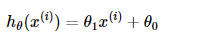其中 i=1,2,...,m表示样本数。

对应的目标函数（代价函数）即为：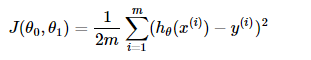下图为 J(θ0+θ1)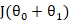与参数θ0θ1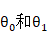的关系的图：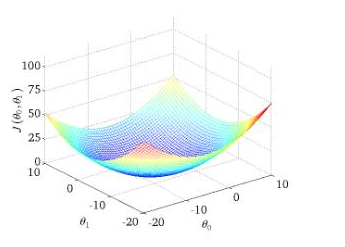批量梯度下降法是最原始的形式，它是指在每一次迭代时使用所有样本来进行梯度的更新。从数学上理解如下：

1. 对目标函数求偏导：其中 i=1,2,...,m表示样本数， j=0,表示特征数，这里我们使用了偏置项x0(i)=1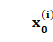1. 每次迭代对参数进行更新：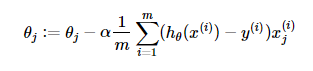注意这里更新时存在一个求和函数，即为对所有样本进行计算处理，可与下文SGD法进行比较。
伪代码形式为：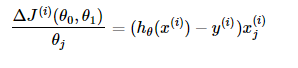其中α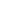表示学习率，一般根据经验进行设置。

优点：
（1）一次迭代是对所有样本进行计算，此时利用矩阵进行操作，实现了并行。
（2）由全数据集确定的方向能够更好地代表样本总体，从而更准确地朝向极值所在的方向。当目标函数为凸函数时，BGD一定能够得到全局最优。
缺点：
（1）当样本数目m很大时，每迭代一步都需要对所有样本计算，训练过程会很慢。
从迭代的次数上来看，BGD迭代的次数相对较少。其迭代的收敛曲线示意图可以表示如下：

梯度下降法不同于批量梯度下降，随机梯度下降是每次迭代使用一个样本来对参数进行更新。使得训练速度加快。
样本的目标函数为：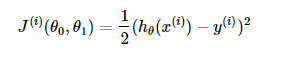1. 对目标函数求偏导：1. 参数更新：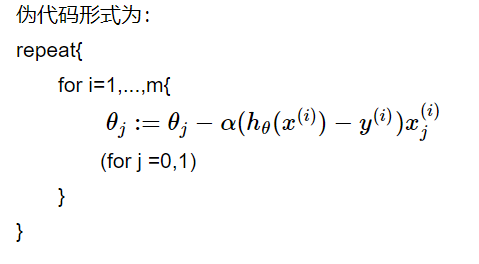注意，这里不再有求和符号

伪代码形式为：优点：
（1）由于不是在全部训练数据上的损失函数，而是在每轮迭代中，随机优化某一条训练数据上的损失函数，这样每一轮参数的更新速度大大加快。
缺点：
（1）准确度下降。由于即使在目标函数为强凸函数的情况下，SGD仍旧无法做到线性收敛。
（2）可能会收敛到局部最优，由于单个样本并不能代表全体样本的趋势。
（3）不易于并行实现。

小批量梯度下降是对批量梯度下降以及随机梯度下降的一个折中办法。其思想是：每次迭代 使用 ** batch_size** 个样本来对参数进行更新。
这里我们假设batchsize=10 ，样本数 m=1000
伪代码形式为：优点：
（1）通过矩阵运算，每次在一个batch上优化神经网络参数并不会比单个数据慢太多。
（2）每次使用一个batch可以大大减小收敛所需要的迭代次数，同时可以使收敛到的结果更加接近梯度下降的效果。(比如上例中的30W，设置batch_size=100时，需要迭代3000次，远小于SGD的30W次)
（3）可实现并行化。
缺点：
（1）batch_size的不当选择可能会带来一些问题。

batcha_size的选择带来的影响：
（1）在合理地范围内，增大batch_size的好处：
a. 内存利用率提高了，大矩阵乘法的并行化效率提高。
b. 跑完一次 epoch（全数据集）所需的迭代次数减少，对于相同数据量的处理速度进一步加快。
c. 在一定范围内，一般来说 Batch_Size 越大，其确定的下降方向越准，引起训练震荡越小。

（2）盲目增大batch_size的坏处：
a. 内存利用率提高，但是内存容量可能不足。
b. 跑完一次 epoch（全数据集）所需的迭代次数减少，要想达到相同的精度，其所花费的时间大大增加了，从而对参数的修正也就显得更加缓慢。
c. Batch_Size 增大到一定程度，其确定的下降方向已经基本不再变化。

牛顿迭代法

牛顿法的基本思想是利用迭代点处的一阶导数(梯度)和二阶导数(Hessen矩阵)对目标函数进行二次函数近似，然后把二次模型的极小点作为新的迭代点，并不断重复这一过程，直至求得满足精度的近似极小值。牛顿法的速度相当快，而且能高度逼近最优值。牛顿法分为基本的牛顿法和全局牛顿法。

• 牛顿法计算函数零点

首先，选择一个接近函数f(x)零点的x0，计算相应的f(x0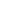) 和切线斜率 f'(x0)（这里 f'表示函数f的导数）。然后我们计算穿过点 (x0,f(x0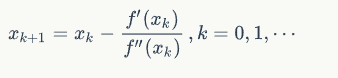))并且斜率为f'(x0) 的直线和x轴的交点的x坐标，也就是求如下方程的解：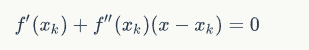我们将新求得的点的x坐标命名为x_1，通常 x_1会比x_{0}更接近方程 f(x)=0的解。因此我们现在可以利用x_1开始下一轮迭代。迭代公式可化简为如下所示：已有证明牛顿迭代法的二次收敛必须满足以下条件：

1. f'(x)≠ 0};
2. 对于所有x ∈I，其中 I为区间[α − r, α + r]，且x0在区间I内，即 r>=|a-x0|

3、对于所有x ∈I，f''(x)是连续的;

4、x0足够接近根 α。

• 牛顿法求解最优化问题
1. 考虑一维的简单情形

简单情形N=1（此时目标函数f(X)  变为f(x) .牛顿法的基本思想是：在现有极小点估计值的附近对f(x)做二阶泰勒展开，进而找到极小点的下一个估计值。

f(x) 为当前的极小点估计值，则:表示f(x) xk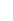附近的二阶泰勒展开式（其中略去了关于x-xk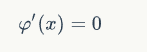的高阶无穷小项）。

由于求的是最值，由极值必要条件可知， φ(x)应满足：即：解得：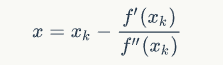于是，若给定初始值x0,则可以构造如下的迭代格式:产生序列{xk}来逼近f(x)的极小点。在一定条件下，{xk}可以收敛到f(x)的极小点。

1. 对于N>1的情形，二阶泰勒展开式可以做推广，此时: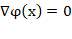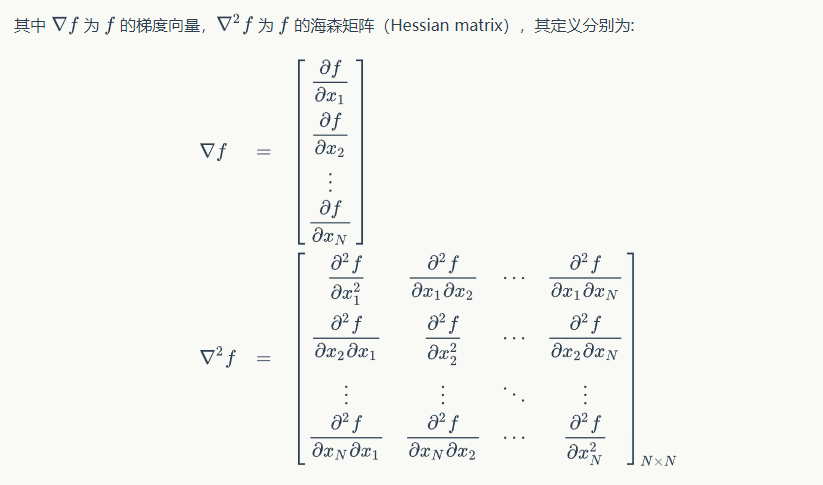注意：

1∇f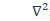和∇2f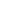中的元素均为关于x的函数，以下分别将其简记为g和H。

2、f(xk)和∇2f(xk)表示将x取为xk后得到的实值向量和矩阵，以下分别将其简记为gkHk同样地，由于是求极小点，极值必要条件要求它为φ（x）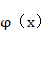的驻点，即:∇φx=0对泰勒展开使用梯度算子得: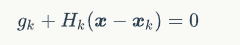进一步，若矩阵HK非奇异，则可解得:于是，若给定初始值x0，则同样可以构造出迭代格式: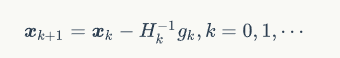这就是原始的牛顿迭代法，其迭代格式中的搜索方向dk=-Hk-1gk称为牛顿方向.

牛顿法的优点：

当目标函数是二次函数时，由于二次泰勒展开函数与原目标函数不是近似而是完全相同的二次式，海森矩阵退化成一个常数矩阵，从任一初始点出发，利用xk+1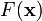的迭代式，只需一步迭代即可达到x的极小点x*，因此牛顿法是一种具有二次收敛性的算法。对于非二次函数，若函数的二次性态较强，或迭代点已进入极小点的领域，则其收敛速度也是很快的，这是牛顿法的主要优点.

牛顿法的缺点：

但原始牛顿法由于迭代公式中没有步长因子，而是定步长迭代，对于非二次目标函数来说，有时会使函数值上升，即出现f(xk+1)>f(xk)的情况，这表明原始牛顿法不能保证函数值稳定地下降.在严重的情况下甚至可能造成迭代点列{xk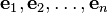}的发散而导致计算失败.

坐标下降法

坐标下降优化方法是一种非梯度优化算法。为了找到一个函数的局部极小值，在每次迭代中可以在当前点处沿一个坐标方向进行一维搜索。在整个过程中循环使用不同的坐标方向。一个周期的一维搜索迭代过程相当于一个梯度迭代。

算法描述：

坐标下降法基于的思想是多变量函数可以通过每次沿一个方向优化来获取最小值。与通过梯度获取最速下降的方向不同，在坐标下降法中，优化方向从算法一开始就予以固定。例如，可以选择线性空间的一组基作为搜索方向。 在算法中，循环最小化各个坐标方向上的目标函数值。亦即，如果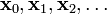已给定，那么，的第个维度为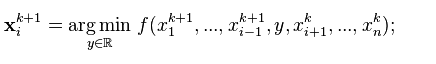因而，从一个初始的猜测值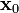以求得函数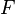的局部最优值，可以迭代获得的序列。

通过在每一次迭代中采用一维搜索，可以很自然地获得不等式可以知道，这一序列与最速下降具有类似的收敛性质。如果在某次迭代中，函数得不到优化，说明一个驻点已经达到。

这一过程可以用下图表示。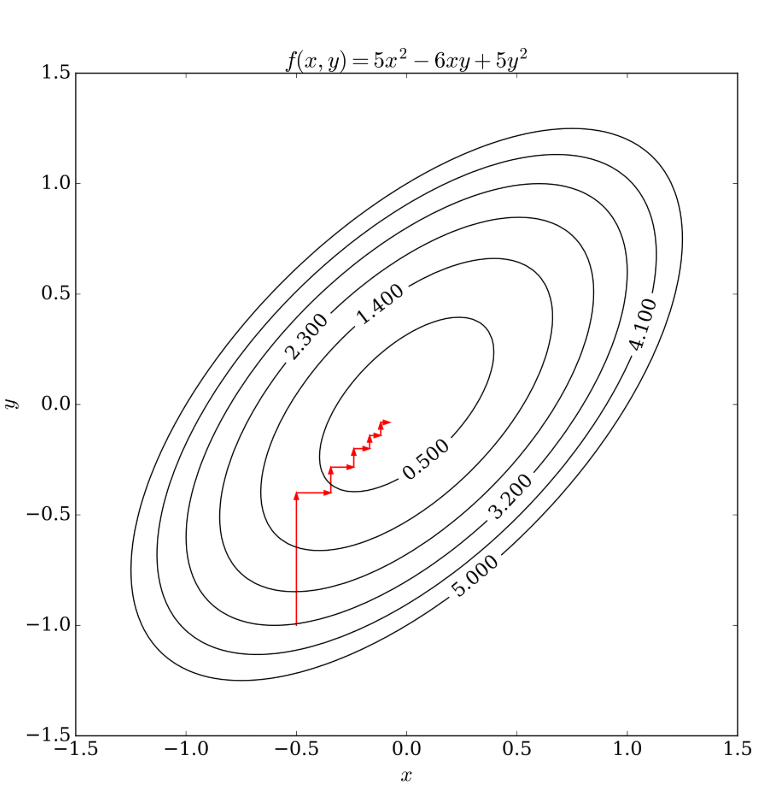梯度下降法、牛顿法、和坐标下降法优缺点对比

1、梯度下降法

梯度下降法实现简单，当目标函数是凸函数时，梯度下降法的解是全局解。一般情况下，其解不保证是全局最优解，梯度下降法的速度也未必是最快的。

梯度下降法的优化思想：用当前位置负梯度方向作为搜索方向，因为该方向为当前位置的最快下降方向，所以也被称为是“最速下降法”。最速下降法越接近目标值，步长越小，前进越慢。

缺点：

靠近极小值时收敛速度减慢，求解需要很多次的迭代；

直线搜索时可能会产生一些问题；

可能会“之字形”地下降。

2、牛顿法

牛顿法最大的特点就在于它的收敛速度很快。

优点：二阶收敛，收敛速度快；

缺点：

牛顿法是一种迭代算法，每一步都需要求解目标函数的Hessian矩阵的逆矩阵，计算比较复杂。

牛顿法收敛速度为二阶，对于正定二次函数一步迭代即达最优解。

牛顿法是局部收敛的，当初始点选择不当时，往往导致不收敛；

二阶海塞矩阵必须可逆，否则算法进行困难。

关于牛顿法和梯度下降法的效率对比：

从本质上去看，牛顿法是二阶收敛，梯度下降是一阶收敛，所以牛顿法就更快。如果更通俗地说的话，比如你想找一条最短的路径走到一个盆地的最底部，梯度下降法每次只从你当前所处位置选一个坡度最大的方向走一步，牛顿法在选择方向时，不仅会考虑坡度是否够大，还会考虑你走了一步之后，坡度是否会变得更大。所以，可以说牛顿法比梯度下降法看得更远一点，能更快地走到最底部。（牛顿法目光更加长远，所以少走弯路；相对而言，梯度下降法只考虑了局部的最优，没有全局思想。）

3、坐标下降法求最值问题和梯度下降法对比

1. 坐标下降法在每次迭代中在当前点处沿一个坐标方向进行一维搜索 ，固定其他的坐标方向，找到一个函数的局部极小值。而梯度下降总是沿着梯度的负方向求函数的局部最小值。
2. 坐标下降优化方法是一种非梯度优化算法。在整个过程中依次循环使用不同的坐标方向进行迭代，一个周期的一维搜索迭代过程相当于一个梯度下降的迭代。
3. 梯度下降是利用目标函数的导数来确定搜索方向的，该梯度方向可能不与任何坐标轴平行。而坐标下降法法是利用当前坐标方向进行搜索，不需要求目标函数的导数，只按照某一坐标方向进行搜索最小值。
4. 两者都是迭代方法，且每一轮迭代，都需要O(mn)的计算量(m为样本数，n为系数向量的维度)

实验效果对比

1、线性问题拟合y = 3*x1 + 4*x2

数据集：代码生成的1000个样本点 X

对比算法：

1、随机梯度下降

2、批量梯度下降

3、小批量梯度下降

对别内容：

1、生成参数

2、算法迭代次数

3、算法运行时间

实验结果：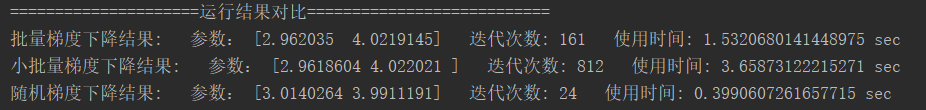1、非线性问题拟合最优化

已知函数f(x1,x2)=(1 - x1)2 + 100 * (x2 - x12)2

是光滑的凸函数，且只有一个最小值点f(1,1)=0

对比算法：

1、随机梯度下降

2、牛顿法

对别内容：

1、精度损失

2、最优点坐标

3、算法运行时间

实验结果：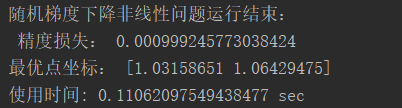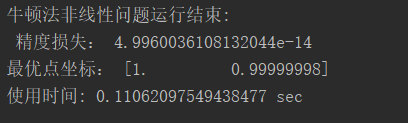代码实现：

from mpl_toolkits.mplot3d import axes3d
import matplotlib.pyplot as plt
from matplotlib import cm
import numpy as np
import random
import time
def print_Chart():
# fig = plt.figure()
fig = plt.figure()
ax.set_xlabel('X')
ax.set_ylabel('Y')
ax.set_zlabel('Z')
# Make data
X = np.linspace(-2, 2, 100)
Y = np.linspace(-2,2, 100)
X, Y = np.meshgrid(X, Y)
Z =(1-X)**2+(X-Y**2)**2*100
# Plot the surface
ax.plot_surface(X, Y, Z, color='g')
plt.show()

def cal_rosenbrock(x1, x2):
"""
计算rosenbrock函数的值
:param x1:
:param x2:
:return:
"""
return (1 - x1) ** 2 + 100 * (x2 - x1 ** 2) ** 2

def cal_rosenbrock_prax(x1, x2):
"""
对x1求偏导
"""
return -2 + 2 * x1 - 400 * (x2 - x1 ** 2) * x1

def cal_rosenbrock_pray(x1, x2):
"""
对x2求偏导
"""
return 200 * (x2 - x1 ** 2)

'''
梯度下降
最小值为0
默认初试点(0,0)
:param max_iter_count:
:param step_size:
:param x1:
:param x2:
:return:
'''
pre_x = np.array([x1,x2])
loss = 10
iter_count = 0
while loss > 0.001 and iter_count < max_iter_count:
error = np.zeros((2,), dtype=np.float32)
error = cal_rosenbrock_prax(pre_x, pre_x)
error = cal_rosenbrock_pray(pre_x, pre_x)

for j in range(2):
pre_x[j] -= step_size * error[j]

loss = cal_rosenbrock(pre_x, pre_x)  # 目标值为0

print("iter_count: ", iter_count, "the loss:", loss)
iter_count += 1
return loss,pre_x

def h(x1=0.0,x2=0.0,r=2,c=2):
_h=np.zeros((r,c),dtype=np.float32)
_h=2+1200*x1**2-400*x2
_h=-400*x1
_h=_h
_h=200
return _h

def g(x1=0.0,x2=0.0):
return np.array([cal_rosenbrock_prax(x1,x2),cal_rosenbrock_pray(x1,x2)])

def newton(max_iter_count=100000,x1=0.0,x2=0.0):
pre_x = np.array([x1,x2])
loss = 10
iter_count = 0
while loss > 0.001 and iter_count < max_iter_count:
h_inv=np.linalg.inv(h(x1=pre_x,x2=pre_x))
_g=np.matrix(g(pre_x,pre_x).reshape([2,1]))
t=h_inv*_g
for i in range(t.size):
pre_x[i]-=t[i]
loss = cal_rosenbrock(pre_x, pre_x)  # 目标值为0
print("iter_count: ", iter_count, "the loss:", loss)
iter_count += 1
return loss,pre_x

'''
BGD（批量梯度下降法）
'''
def gen_line_data(sample_num=1000):
"""
y = 3*x1 + 4*x2
:return:
"""
x1 = np.linspace(0, 9, sample_num)
x2 = np.linspace(4, 13, sample_num)
x = np.concatenate(([x1], [x2]), axis=0).T
y = np.dot(x, np.array([3, 4]).T)  # y 列向量
return x, y

def bgd(samples, y, step_size=0.01, max_iter_count=10000):
'''
批量梯度下降
:param samples:
:param y:
:param step_size:
:param max_iter_count:
:return:
'''
sample_num, dim = samples.shape
y = y.flatten()
w = np.ones((dim,), dtype=np.float32)
loss = 10
iter_count = 0
while loss > 0.001 and iter_count < max_iter_count:
loss = 0 #每次迭代从新计算受损失值
error = np.zeros((dim,), dtype=np.float32)
'''
遍历所有样本后更新一次参数
'''
for i in range(sample_num):
predict_y = np.dot(w.T, samples[i])
for j in range(dim):
error[j] += (y[i] - predict_y) * samples[i][j]

for j in range(dim):
w[j] += step_size * error[j] / sample_num

for i in range(sample_num):
predict_y = np.dot(w.T, samples[i])
error = (1 / (sample_num * dim)) * np.power((predict_y - y[i]), 2)
loss += error

print("iter_count: ", iter_count, "the loss:", loss)
iter_count += 1
return w,iter_count

def mbgd(samples, y, step_size=0.01, max_iter_count=10000, batch_size=0.2):
"""
:param samples:
:param y:
:param step_size:
:param max_iter_count:
:param batch_size:
:return:
"""
sample_num, dim = samples.shape
y = y.flatten()
w = np.ones((dim,), dtype=np.float32)
# batch_size = np.ceil(sample_num * batch_size)
loss = 10
iter_count = 0
while loss > 0.001 and iter_count < max_iter_count:
loss = 0
error = np.zeros((dim,), dtype=np.float32)

# batch_samples, batch_y = select_random_samples(samples, y,
# batch_size)

index = random.sample(range(sample_num),
int(np.ceil(sample_num * batch_size)))
batch_samples = samples[index]
batch_y = y[index]

for i in range(len(batch_samples)):
predict_y = np.dot(w.T, batch_samples[i])
for j in range(dim):
error[j] += (batch_y[i] - predict_y) * batch_samples[i][j]

for j in range(dim):
w[j] += step_size * error[j] / sample_num

for i in range(sample_num):
predict_y = np.dot(w.T, samples[i])
error = (1 / (sample_num * dim)) * np.power((predict_y - y[i]), 2)
loss += error

print("iter_count: ", iter_count, "the loss:", loss)
iter_count += 1
return w,iter_count

def sgd(samples, y, step_size=0.01, max_iter_count=10000):
"""
随机梯度下降法,每读取一次样本后都对参数进行更新
:param samples: 样本
:param y: 结果value
:param step_size: 每一接迭代的步长
:param max_iter_count: 最大的迭代次数
:param batch_size: 随机选取的相对于总样本的大小
:return:
"""
sample_num, dim = samples.shape
y = y.flatten()
w = np.ones((dim,), dtype=np.float32)
loss = 10
iter_count = 0
while loss > 0.001 and iter_count < max_iter_count:
loss = 0
error = np.zeros((dim,), dtype=np.float32)
for i in range(sample_num):
predict_y = np.dot(w.T, samples[i])
for j in range(dim):
'''
每次读取一个样本后对参数进行更新，
下一个样本使用更新后的参数进行估计
'''
error[j] += (y[i] - predict_y) * samples[i][j]
w[j] += step_size * error[j] / sample_num

for i in range(sample_num):
predict_y = np.dot(w.T, samples[i])
error = (1 / (sample_num * dim)) * np.power((predict_y - y[i]), 2)
loss += error

print("iter_count: ", iter_count, "the loss:", loss)
iter_count += 1
return w,iter_count

'''
在使用坐标下降算法
对x1求偏导为0时，无法显示的用x2来表示x1
因此该方程不适用于坐标下降算法

'''
# def coordinate_descent(max_iter_count=100000,x1=0.0,x2=0.0):
#
#     def cordinate_x1(x1=0.0,x2=0.0):
#
#     def cordinate_x1(x1=0.0,x2=0.0):
#
#     pre_x = np.array([x1,x2])
#     loss = 10
#     iter_count = 0
#     while loss > 0.001 and iter_count < max_iter_count:
#         h_inv=np.linalg.inv(h(x1=pre_x,x2=pre_x))
#         _g=np.matrix(g(pre_x,pre_x).reshape([2,1]))
#         t=h_inv*_g
#         for i in range(t.size):
#             pre_x[i]-=t[i]
#         loss = cal_rosenbrock(pre_x, pre_x)  # 目标值为0
#         print("iter_count: ", iter_count, "the loss:", loss)
#         iter_count += 1
#     return loss,pre_x

if __name__ == '__main__':
# print_Chart()

# 批量梯度下降，线性问题
samples, y = gen_line_data()
time1=time.time()
w1,iter_count1 = bgd(samples, y)
cost_time1=time.time()-time1
print('批量梯度下降结果:','  参数：',w1,'  迭代次数:',iter_count1,'  使用时间:',cost_time1,'sec')

time2=time.time()
w2,iter_count2=mbgd(samples,y)
cost_time2=time.time()-time2
print('小批量梯度下降结果:','  参数：',w2,'  迭代次数:',iter_count2,'  使用时间:',cost_time2,'sec')

time3=time.time()
w3,iter_count3=sgd(samples,y)
cost_time3=time.time()-time3

print('=====================运行结果对比===========================')
print('批量梯度下降结果:','  参数：',w1,'  迭代次数:',iter_count1,'  使用时间:',cost_time1,'sec')
print('小批量梯度下降结果:','  参数：',w2,'  迭代次数:',iter_count2,'  使用时间:',cost_time2,'sec')
print('随机梯度下降结果:','  参数：',w3,'  迭代次数:',iter_count3,'  使用时间:',cost_time3,'sec')

'''
非线性问题
'''
# 梯度下降 非线性问题
time4=time.time()
cost_time4=time.time()-time4
print('随机梯度下降非线性问题运行结束:\n','精度损失:',loss,'\n最优点坐标:',coordinate, '\n使用时间:',cost_time4,'sec')

# 牛顿法 非线性问题
time5=time.time()
loss,pre_x=newton()
cost_time5=time.time()-time4
print('牛顿法非线性问题运行结束:\n','精度损失:',loss,'\n最优点坐标:',pre_x, '\n使用时间:',cost_time5,'sec')
展开全文深度学习 python
• 高斯--牛顿迭代法基本思想是使用泰勒级数展开式去近似地代替非线性回归模型，然后通过多次迭代，多次修正回归系数，使回归系数不断逼近非线性回归模型的最佳回归系数，最后使原模型的残差平方和达到最小。...梯度下降法
• 梯度下降法算法总结

千次阅读 多人点赞 2020-02-25 23:33:55算法 python 计算机视觉 机器学习 人工智能
• 迭代次数:%d' % (alpha, x1, x2, f2(x1,x2), iter_num)) plt.show() 得到的结果以及显示为： 牛顿迭代法的原理 牛顿迭代法是一种线性化方法，基本思想就是将非线性方程f(x)=0逐步归结为某种线性方程来求解。...python 机器学习
• 迭代法 python

2020-12-20 07:25:59
详解迭代器的使用 | 手把手教你入门Python之八十上一篇：自定义异常 | 手把手教你入门Python之七十九下一篇：生成器 | 手把手教你入门Python之八十一本文来自于千锋教育在阿里云开发者社区学习中心上线课程《Python...python的迭代
• 下降法的思想

2018-12-06 22:16:47
假设目标函数为： F(x) = f(x)'f(x). 最速下降法： 对目标函数F(x)，在迭代点处，采用一阶泰勒展开近似，然后把满足近似模型极小值的点，作为新的迭代点。...这两种方法的基本思想，都是对目标函数F...
• 梯度下降算法原理讲解——机器学习

万次阅读 多人点赞 2019-01-21 20:27:48
详细来讲讲梯度下降算法的原理，感受数学和程序的魅力吧！！梯度下降
• 多线性方程组(张量)迭代算法的原理请看这里：原理部分请留言，不方便公开分享import numpy as npimport time1.1 Gauss-Seidel迭代算法def GaussSeidel_tensor_V2(A,b,Delta,m,n,M):start=time.perf_counter()find=0X...
• ISTA算法和FISTA算法是求解线性逆问题的经典方法，隶属于梯度类算法，也常用于压缩感知重构算法中，隶属于梯度类算法，这次将这2中算法原理做简单分析，并给出matlab仿真实验，通过实验结果来验证算法性能。...算法 人工智能 线性代数
• 牛顿迭代法又称为牛顿-拉弗森方法，实际上是由牛顿、拉弗森各自独立提出来的。牛顿-拉弗森方法提出来的思路就是利用切线是曲线的线性逼近这个思想，如下图所示： 随便找一个曲线上的A点（为什么随便找，根据...算法 机器学习 人工智能 python
• 1. 迭代法的收敛速度 迭代过程的收敛速度，是指迭代误差的下降速度。迭代法的收敛速度一般用收敛阶来描述。 定义2：对于收敛的迭代法xk+1=φ(xk),(k=1,2,⋯ )x_{k+1}=\varphi(x_k),(k=1,2,\cdots)xk+1​=φ(xk​),...
• 梯度下降法是一个最优化算法，通常也称为最速下降法。 假设f(x)是一座山，站在半山腰，往x方向走1米，高度上升0.4米，也就是说x方向上的偏导是 0.4；往y方向走1米，高度上升0.3米，也就是说y方向上的偏导是 0.3；...
• 其它机器学习、深度学习算法的全面系统讲解可以阅读《机器学习-原理、算法与应用》...优化算法中，梯度下降法是最简单、最常见的一种，在深度学习的训练中被广为使用。在本文中，SIGAI 将为大家系统的讲述梯度下降法...梯度下降算法
• 迭代法

2018-10-31 10:48:11
迭代算法是用计算机解决问题的一种基本方法，它利用计算机运算速度快、适合做重复性操作的特点，让计算机对一组指令(或一定步骤)进行重复执行，在每次执行这组指令(或这些步骤)时，都从变量的原值推出它的一个...
• 监督学习： 训练集是一组有输入，且知道正确输出的数据。 训练的过程即通过这组数据，构建一个函数，使在同样的输入下，这个函数的输出与真实的输出之间的差距尽量小。...梯度下降方法： 如图所示，即从
• 最小二乘法 基本公式： 考虑超定方程组（超定...梯度下降法是一个最优化算法，常用于机器学习和人工智能当中用来递归性地逼近最小偏差模型。 顾名思义，梯度下降法的计算过程就是沿梯度下降的方向求解极小值（也可以沿
• 梯度下降法

千次阅读 2019-03-03 23:15:13
梯度下降法基本思想可以类比为一个下山的过程。假设这样一个场景：一个人被困在山上，需要从山上下来(i.e. 找到山的最低点，也就是山谷)。但此时山上的浓雾很大，导致可视度很低。因此，下山的路径就无法确定，他...
• 更新就是优化器的更新策略，每个不同的优化器会采取不同的策略去更新参数的值，这里策略通常是梯度下降。 在展开讨论之前，先明确下面几个基本概念： 导数：函数在指定坐标轴上的变化率 方向导数：指定方向上的变化...机器学习 深度学习 python
• 最优化方法——最速下降法，阻尼牛顿，共轭梯度 目录 最优化方法——最速下降法，阻尼牛顿，共轭梯度 1、不精确一维搜素 1.1 Wolfe-Powell 准则 2、不精确一维搜索算法计算步骤 3、最速下降法 3.1 ...最优化方法 最速下降法 共轭梯度法
•人工智能 推荐系统 python
• 梯度下降法基本思想可以类比为一个下山的过程。假设这样一个场景：一个人被困在山上，需要从山上下来(i.e.找到山的最低点，也就是山谷)。但此时山上的浓雾很大，导致可视度很低。因此，下山的路径就无法确定，他...梯度下降算法
• 梯度下降算法简明教程

千次阅读 2018-10-21 20:03:52
最早接触梯度下降算法是在学习逻辑回归（Logistic Regression），对于权重的迭代更新。当然运用梯度算法的地方远不止逻辑回归，该方法思路简单但适用范围很广，简单的线性回归（Linear Regression），以及最近在看的...梯度下降算法 一阶泰勒展开式
• 【IT168 评论】本节尝试独立于机器学习算法, 单纯地来讲梯度下降算法 [Gradient Descent, GD], 以使梯度下降更具一般性.开始之前, 先放 2 个基本概念, 带着这 2 个认识, 在几乎不具备机器学习知识的前提下, 应该也能...
• 李航老师在《统计学习方法》中将机器学习的三要素总结为：模型、策略和算法。其大致含义如下： 模型：其实就是机器学习训练的过程中所要学习的条件概率分布或者决策函数。 策略：就是使用一种什么样的评价，度量...梯度下降 共轭梯度下降 机器学习算法...